CSDN视频网址：http://edu.csdn.net/lecturer/144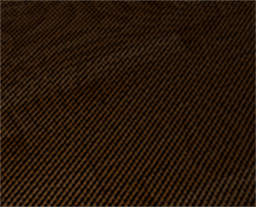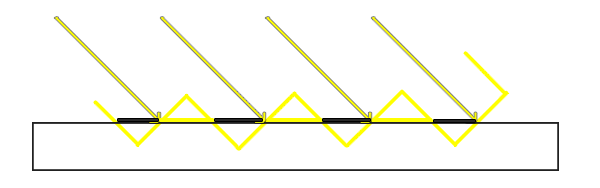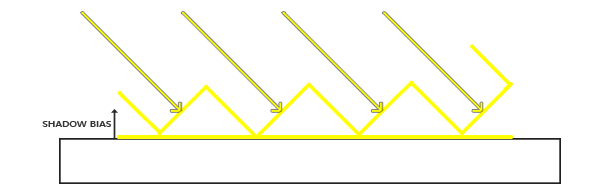float bias = 0.005;
float shadow = currentDepth - bias > closestDepth  ? 1.0 : 0.0;

float bias = max(0.05 * (1.0 - dot(normal, lightDir)), 0.005);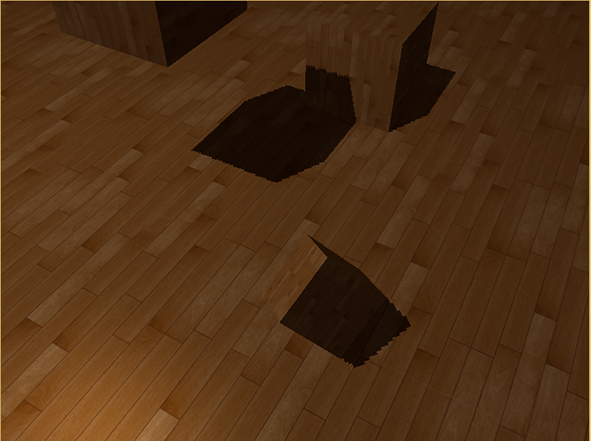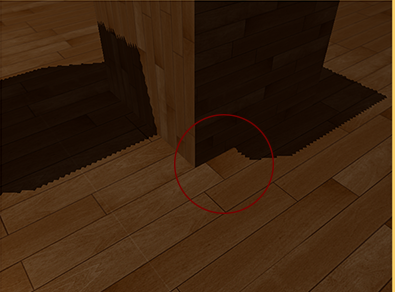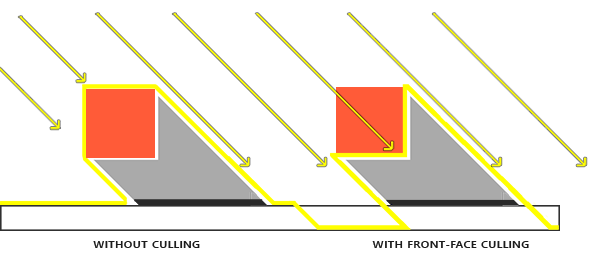glCullFace(GL_FRONT);
RenderSceneToDepthMap();
glCullFace(GL_BACK); // 不要忘记设回原先的culling face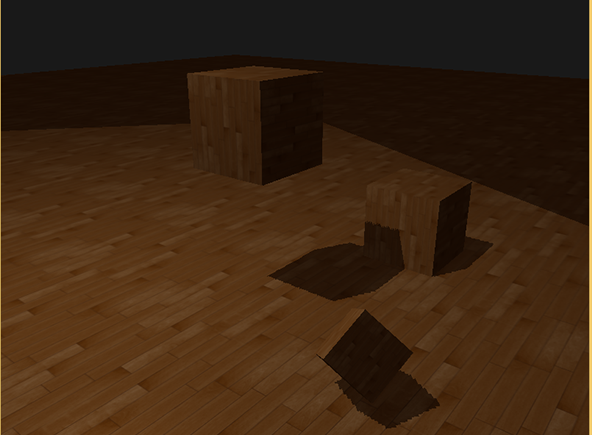glTexParameteri(GL_TEXTURE_2D, GL_TEXTURE_WRAP_S, GL_CLAMP_TO_BORDER);
glTexParameteri(GL_TEXTURE_2D, GL_TEXTURE_WRAP_T, GL_CLAMP_TO_BORDER);
GLfloat borderColor[] = { 1.0, 1.0, 1.0, 1.0 };
glTexParameterfv(GL_TEXTURE_2D, GL_TEXTURE_BORDER_COLOR, borderColor);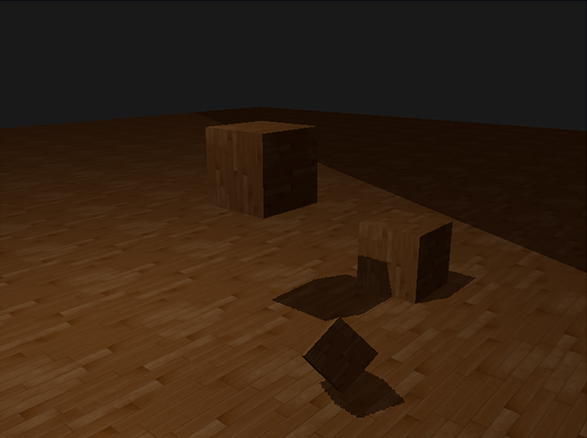float ShadowCalculation(vec4 fragPosLightSpace)
{
[...]
if(projCoords.z > 1.0)

}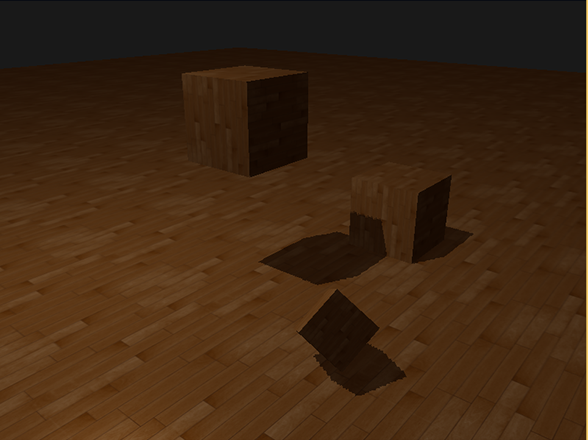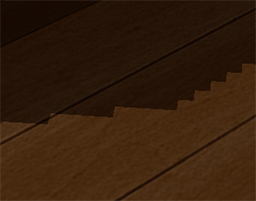float shadow = 0.0;
vec2 texelSize = 1.0 / textureSize(shadowMap, 0);
for(int x = -1; x <= 1; ++x)
{
for(int y = -1; y <= 1; ++y)
{
float pcfDepth = texture(shadowMap, projCoords.xy + vec2(x, y) * texelSize).r;
shadow += currentDepth - bias > pcfDepth ? 1.0 : 0.0;
}
}
shadow /= 9.0;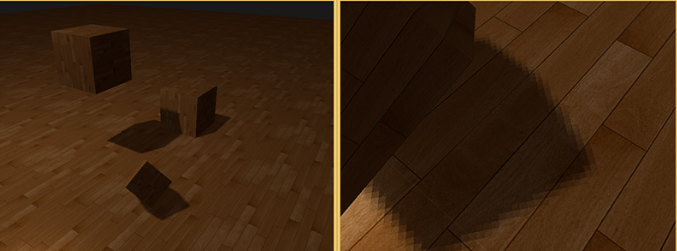#version 330 core
layout (location = 0) in vec3 position;
layout (location = 1) in vec3 normal;
layout (location = 2) in vec2 texCoords;

out VS_OUT {
vec3 FragPos;
vec3 Normal;
vec2 TexCoords;
vec4 FragPosLightSpace;
} vs_out;

uniform mat4 projection;
uniform mat4 view;
uniform mat4 model;
uniform mat4 lightSpaceMatrix;

void main()
{
gl_Position = projection * view * model * vec4(position, 1.0f);
vs_out.FragPos = vec3(model * vec4(position, 1.0));
vs_out.Normal = transpose(inverse(mat3(model))) * normal;
vs_out.TexCoords = texCoords;
vs_out.FragPosLightSpace = lightSpaceMatrix * vec4(vs_out.FragPos, 1.0);
}

#version 330 core
out vec4 FragColor;

in VS_OUT {
vec3 FragPos;
vec3 Normal;
vec2 TexCoords;
vec4 FragPosLightSpace;
} fs_in;

uniform sampler2D diffuseTexture;

uniform vec3 lightPos;
uniform vec3 viewPos;

{
// perform perspective divide
vec3 projCoords = fragPosLightSpace.xyz / fragPosLightSpace.w;
// Transform to [0,1] range
projCoords = projCoords * 0.5 + 0.5;
// Get closest depth value from light's perspective (using [0,1] range fragPosLight as coords)
// Get depth of current fragment from light's perspective
float currentDepth = projCoords.z;
// Calculate bias (based on depth map resolution and slope)
vec3 normal = normalize(fs_in.Normal);
vec3 lightDir = normalize(lightPos - fs_in.FragPos);
float bias = max(0.05 * (1.0 - dot(normal, lightDir)), 0.005);
// Check whether current frag pos is in shadow
// float shadow = currentDepth - bias > closestDepth  ? 1.0 : 0.0;
// PCF
vec2 texelSize = 1.0 / textureSize(shadowMap, 0);
for(int x = -1; x <= 1; ++x)
{
for(int y = -1; y <= 1; ++y)
{
float pcfDepth = texture(shadowMap, projCoords.xy + vec2(x, y) * texelSize).r;
shadow += currentDepth - bias > pcfDepth  ? 1.0 : 0.0;
}
}

// Keep the shadow at 0.0 when outside the far_plane region of the light's frustum.
if(projCoords.z > 1.0)

}

void main()
{
vec3 color = texture(diffuseTexture, fs_in.TexCoords).rgb;
vec3 normal = normalize(fs_in.Normal);
vec3 lightColor = vec3(0.4);
// Ambient
vec3 ambient = 0.2 * color;
// Diffuse
vec3 lightDir = normalize(lightPos - fs_in.FragPos);
float diff = max(dot(lightDir, normal), 0.0);
vec3 diffuse = diff * lightColor;
// Specular
vec3 viewDir = normalize(viewPos - fs_in.FragPos);
float spec = 0.0;
vec3 halfwayDir = normalize(lightDir + viewDir);
spec = pow(max(dot(normal, halfwayDir), 0.0), 64.0);
vec3 specular = spec * lightColor;
vec3 lighting = (ambient + (1.0 - shadow) * (diffuse + specular)) * color;

FragColor = vec4(lighting, 1.0f);
}©️2019 CSDN 皮肤主题: 大白 设计师: CSDN官方博客